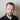# Generating thousands of trading strategies using Frechet distance

March 26, 2020 | strategy

This one will be fun excursion to the land of the selection bias.

We use pattern recognition. There are many ways to calculate patterns, here we’ll use Frechet distance.

Frechet distance is a measure of similarity between curves in mathematics.

My plan:

• generate random 3-point patterns, total of 999
• calculate rolling distance between pattern and price
• buy on threshold for each strategy and plot results

## Generate patterns:

patterns = []
for i in range(0,10):
for k in range(0,10):
for s in range(0,10):
patterns.append([i, k, s])

## Write rolling Frechet:

Install requirements:

pip install frechetdist
from frechetdist import frdist
from pandas import DataFrame

def rolling_frechet(df, pattern):
# for each point of pattern add sequence number:
P = [[(i+1), pattern[i]] for i in range(len(pattern))]
# create some empty points, because pattern will require past data:
out =  * (len(pattern)+1)
# for each data point since we have initial data for Frechet:
for i in range(len(pattern), len(df.index)-1):
try:
# bam, din't even got into math
f = frdist(P, [[(k+1), df.iloc[i-len(pattern)-1+k]] for k in range(len(pattern))])
out.append(f)
except Exception as e:
print(e)
# construct DataFrame
d = DataFrame(out, columns=['fret'], index=df.index)
return d

## Give me the money!

df = get_btc()
df['pct'] = df['close'].pct_change()

# for each pattern
for pattern in patterns:
df['fret'] = rolling_frechet(df['pct'], pattern)
# haha, I'm looking into future here with this threshold:
m = df['fret'].replace([inf, -inf], nan).dropna().mean()
df['signal'] = where(df['fret'] > m, 1, 0)
df['returns'] = df['signal'].shift() * df['pct']
df['cumulative'] = df['returns'].cumsum()
df['cumulative'].plot()
plt.show()

Result:Enjoy, you can write any pattern you like, but this code will choke with lenghty patterns.

## Bonus - example patterns

rectangle = [1, 2, 1, 2, 0]
cup = [6, 3, 2, 1, 1, 1, 2, 3, 6, 0]
zigzag = [1, 7, 2, 8, 0]
head_and_shoulders = [1, 2, 3, 3, 3, 4, 5, 6, 6, 5, 4, 3, 3, 3, 2, 1, 0]
cup = [6, 3, 2, 1, 1, 1, 2, 3, 6, 0]
triangle_symmetric = [1, 8, 2, 7, 3, 6, 5, 4, 0]
triangle_assymetric = [1, 3, 5, 8, 6, 4, 2, 5, 7, 6, 4, 3, 6, 0]Written by Tadas Talaikis